# NCERT Solutions for Chapter 7 Fractions Class 6 MathsChapter Name Fractions NCERT Solutions Class CBSE Class 6 Textbook Name Maths Related Readings NCERT Solutions for Class 6NCERT Solutions for Class 6 Maths Summary of Fractions

Exercise 7.1

Question 1: Write the fraction representing the shaded portion: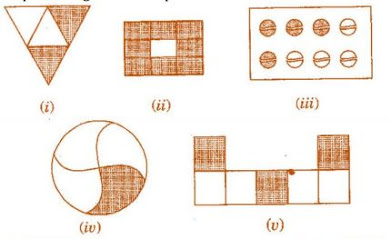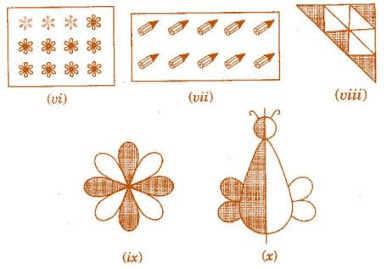Solution

(i) 2/4

(ii) 8/9

(iii) 4/8

(iv) 1/4

(v) 3/7

(vi) 3/12

(vii) 10/10

(viii) 4/9

(ix) 4/8

(x) 1/2

Question 2: Colour the part according to the given fraction: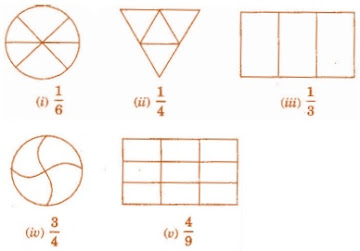Solution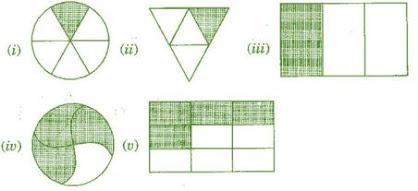Question 3: Identify the error, if any?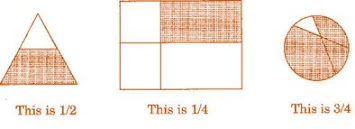Solution

All the figures are not equally divided. For making fractions, it is necessary that figure is to be divided in equal parts.

Question 4: What fraction of a day is 8 hours?

Solution

Since, 1 day = 24 hours.

Therefore, the fraction of 8 hours = 8/24=1/3

Question 5: What fraction of an hour is 40 minutes?

Solution

Since, 1 hour = 60 minutes.

Therefore, the fraction of 40 minutes = 40/60=2/3

Question 6: Arya, Abhimanyu and Vivek shared lunch. Arya has brought two sandwiches, one made of vegetable and one of jam. The other two boys forgot to bring their lunch. Arya agreed to share his sandwiches so that each person will have an equal share of each sandwich.

(a) How can Arya divide his sandwiches so that each person has an equal share?

(b) What part of a sandwich will each boy receive?

Solution

(a) Arya will divide each sandwich into three equal parts and give one part of each sandwich to each one of them.

(b) 1×1/3=1/3

Question 7: Kanchan dyes dresses. She had to dye 30 dresses. She has so far finished 20 dresses. What fraction of dresses has she finished?

Solution

Total number of dresses = 30

Work finished = 20

Fraction of finished work = 20/30=2/3

Question 8: Write the natural numbers from 2 to 12. What fraction of them are prime numbers?

Solution

Natural numbers from 2 to 12:

2, 3, 4, 5, 6, 7, 8, 9, 10, 11, 12

Prime numbers from 2 to 12:

2, 3, 5, 7, 11

Hence, fraction of prime numbers = 5/11

Question 9: Write the natural numbers from 102 to 113. What fraction of them are prime numbers?

Solution

Natural numbers from 102 to 113:

102, 103, 104, 105, 106, 107, 108, 109, 110, 111, 112, 113

Prime numbers from 102 to 113:

103, 107, 109, 113

Hence fraction of prime numbers = 4/12=1/3

Question 10: What fraction of these circles have ‘X’s in them?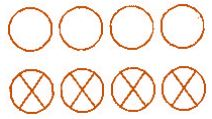Solution

Total number of circles = 8 and number of circles having ‘X’ = 4

Hence, the fraction = 4/8

Question 11: Kristin received a CD player for her birthday. She bought 3 CDs and received 5 others as gifts. What fraction of her total CDs did she buy and what fraction did she receive as gifts?

Solution

Total number of CDs = 3 + 5 = 8

Number of CDs purchased = 3

Fraction of CDs purchased = 3/8

Exercise 7.2

Question 1: Draw number lines and locate the points on them:

(a) 1/2, 1/4, 3/4, 4/4

(b) 1/8, 2/8, 3/8, 7/8

(c) 2/5, 3/5, 8/5, 4/5

Solution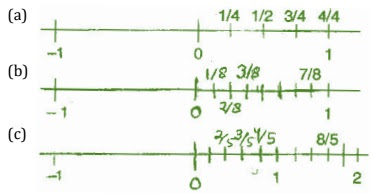Question 2: Express the following fractions as mixed fractions:

(a) 20/3

(b) 11/5

(c)  17/7

(d) 28/5

(e) 19/6

(f) 35/9

Solution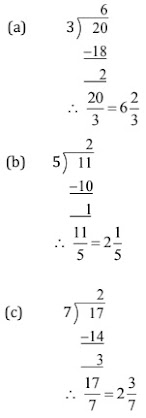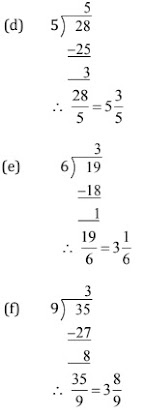Question 3: Express the following as improper fractions:SolutionExercise 7.3

Question 1: Write the fractions. Are all these fractions equivalent: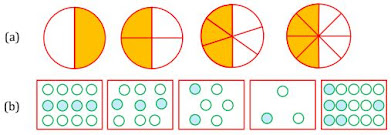Solution

(a) 1/2, 2/4, 3/6, 4/8

Yes, all of these fractions are equivalent.

(b) 4/12, 3/9, 2/6, 1/3, 6/15

No, these fractions are not equivalent

Question 2: Write the fraction and pair up the equivalent fractions to each row: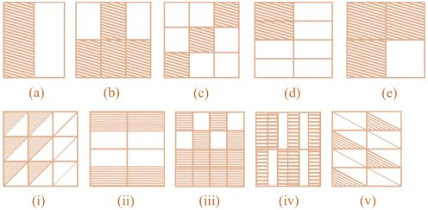Solution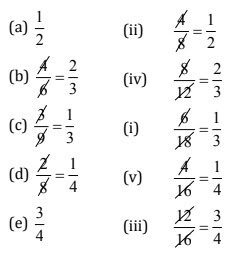Question 3: Replace ロ in each of the following by the correct number:

(a) 2/7=8/ロ

(b) 5/8=10/

(c) 3/20=  /20

(d) 45/60=15/

(e) 18/24=  /4

Solution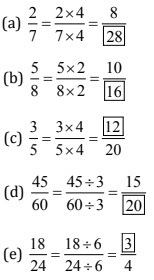Question 4: Find the equivalent fraction of 3/5 having:

(a) denominator 20

(b) numerator 9

(c) denominator 30

(d) numerator 27

Solution

(a) 3/5 = 3×4/5×4 = 12/20

(b) 3/5 = 3×3/5×3 = 9/15

(c) 3/5 = 3×6/5×6 = 18/30

(d) 3/5 = 3×9/5×9 = 27/45

Question 5: Find the equivalent fraction of 36/48 with:

(a) numerator 9

(b) denominator 4

(a) 36/48 = 36÷4/48÷4 = 9/12

(b) 36/48 = 36÷12/48÷12 = 3/4

Question 6: Check whether the given fraction are equivalent:

(a) 5/9, 30/54

(b) 3/10, 12/50

(c) 7/13, 5/11

Solution

(a) 5/9, 30/54 = 5×6/9×6, 30/54 = 30/54, 30/54

Therefore, 5/9,30/54 are equivalent.

(b) 3/10,12/50 = 3×5/10×5, 12/50 = 15/50, 12/50

Therefore, 3/10, 12/50 are not equivalent.

(c) 7/13, 5/11 = 7×11/13×11, 5×13/11×13 = 77/143, 65/143

Therefore, 7/13, 5/11 are not equivalent fraction.

Question 7: Reduce the following fractions to simplest form:

(a) 48/60

(b) 150/60

(c) 84/98

(d) 12/52

(e) 7/28

Solution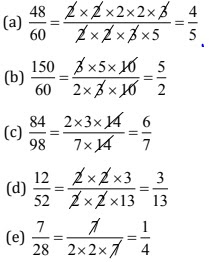Question 8: Ramesh had 20 pencils, Sheelu had 50 pencils and Jamaal had 80 pencils. After 4 months, Ramesh used up 10 pencils, Sheelu used up 25 pencils and Jamaal used up 40 pencils. What fraction did each use up? Check is each has used up an equal fraction of her/his pencils?

Solution

Ramesh:

Total pencils = 20

Pencils used = 10

Fraction = 10/20=1/2

Sheelu:

Total pencils = 50

Pencils used = 25

Fraction = 25/50=1/2

Jamaal:

Total pencils = 80

Pencils used = 40

Fraction = 40/80=1/2

Since, all of them used half of their pencils, therefore each one used up equal fraction of pencils.

Question 9: Match the equivalent fractions and write two more for each:

 Column I Column II 250/400 2/3 180/200 2/5 660/990 1/2 180/360 5/8 220/550 9/10

Solution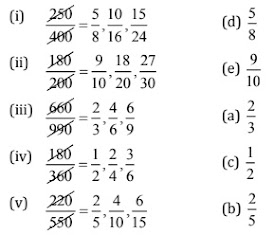Exercise 7.4

Question 1: Write shaded portion as fraction. Arrange them in ascending and descending order using correct sign ‘<’, ‘>’, ‘=’ between the fractions: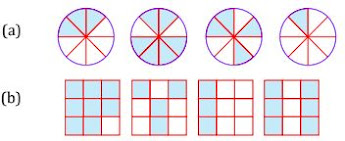(c) Show 2/6, 4/6, 8/6 and 6/6  on the number line. Put appropriate signs between the fractions given:

5/62/6, 3/60, 1/66/6, 8/65/6

Solution

(a) 3/8, 6/8, 4/8, 1/8

Ascending order: 1/8 < 3/8 < 4/8 < 6/8

Descending order: 6/8 > 4/8 > 3/8 > 1/8

(b) 8/9, 4/9, 3/9, 6/9

Ascending order: 3/9 < 4/9 < 6/9 < 8/9

Descending order: 8/9 > 6/9 > 4/9 > 3/9

(c) Number line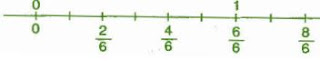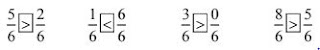Question 2: Compare the fractions and put an appropriate sign:

(a) 3/6 5/6

(b) 1/7 1/4

(c) 4/5 5/5

(d) 3/5 3/7

Solution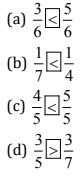Question 3: Make five more each pairs and put appropriate signs.

Solution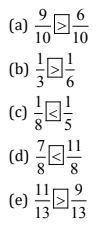Question 4: Look at the figures and write ‘<’ or ‘>’ between the given pairs of fractions: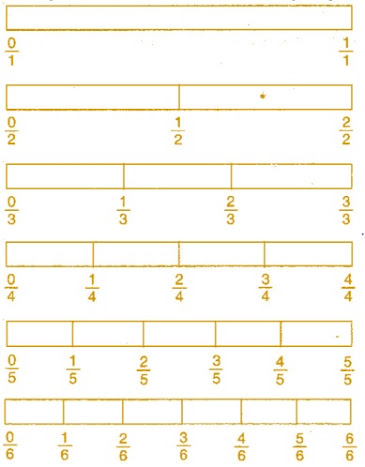(a) 1/61/3

(b) 3/42/6

(c) 2/32/4

(d) 6/63/3

(e) 5/65/5

Make five more such problems and solve them with your friends.

Solution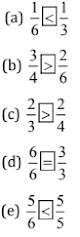Five more such problems:

(a) 1/2ロ3/6

(b) 2/3ロ3/5

(c) 3/4ロ4/6

(d) 5/6ロ2/2

(e) 0/1ロ0/6

Solution: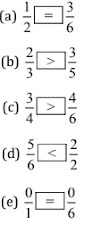Question 5: How quickly can you do this? Fill appropriate sign (<, =, >):

(a) 1/21/5

(b) 2/43/6

(c) 3/52/3

(d) 3/42/8

(e) 3/56/5

(f) 7/93/9

(g) 1/42/8

(h) 6/104/5

(i) 3/47/8

(j) 6/104/5

(k) 5/715/21

Solution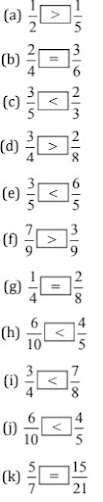Question 6: The following fractions represent just three different numbers. Separate them into three groups of equivalent fractions, by changing each one to its simplest form:

(a) 2/12

(b) 3/15

(c) 8/50

(d) 16/100

(e) 10/60

(f) 15/75

(g) 12/60

(h) 16/96

(i) 12/75

(j) 12/72

(k) 3/18

(l) 4/25

Solution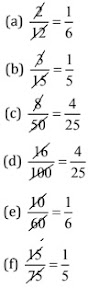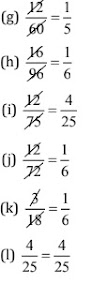Equivalent groups:

I group: 1/5  [(b), (f), (g)]

II group: 1/6  [(a), (e), (h), (j), (k)]

III group: 4/25  [(c), (d), (i), (l)]

Question 7: Find answers to the following. Write and indicate how you solved them:

(a) Is 5/9 equal to 4/5 ?

(b) Is 9/16 equal to 5/9 ?

(c) Is 4/5 equal to 16/20 ?

(d) Is 1/15 equal to 4/30 ?

Solution

(a) 5/9 and 4/5

→ 5×5/9×5 = 25/45 and 4×9/5×9 = 36/45  [∵ L.C.M. of 9 and 5 is 45]

Since, 25/45 ≠ 36/45

Therefore, 5/9 ≠ 4/5

(b) 9/16 and 5/9

→ 9×9/16×9 = 81/144 and 5×16/9×16 = 80/144  [∵ L.C.M. of 16 and 9 is 144]

Since, 81/144 ≠ 80/144

Therefore, 9/16 ≠ 5/16

(c) 4/5 and 16/20

→ 4×20/5×20 = 80/100 and 16×5/20×5 = 80/100  [∵ L.C.M. of 5 and 20 is 100]

Since, 80/100 = 80/100

Therefore, 4/5 = 16/20

(d) 1/15 and 4/30

→ 1×2/15×2 = 2/30 and 4×1/30×1 = 4/30  [∵ L.C.M. of 15 and 30 is 30]

Since, 2/30 ≠ 4/30

Therefore, 1/15 ≠ 4/30

Question 8: Ila read 25 pages of a book containing 100 pages. Lalita read 2/5 of the same book. Who read less?

Solution

Ila read 25 pages out of 100 pages.

Fraction of reading the pages = 25/100 = 1/4th  part of book

Lalita read 2/5th part of book = 40/100 pages

Since 1/4 < 2/5

Question 9: Rafiq exercised for 3/6 of an hour, while Rohit exercised for 3/4 of an hour. Who exercised for a longer time?

Solution

Rafiq exercised 3/6 of an hour.

Rohit exercised 3/4 of an hour.

Since 3/4 > 3/6

Therefore, Rohit exercised for a longer time.

Question 10: In a class A of 25 students, 20 passed in first class; in another class B of 30 students, 24 passed in first class. In which class was a greater fraction of students getting first class?

Solution

In class A, 20 passed out of 25, i.e., 20/25 = 4/5

In class B, 24 passed out of 30, i.e., 24/30 = 4/5

Hence, each class have same fraction of student getting first class.

Exercise 7.5

Question 1: Write the fractions appropriately as additions or subtractions: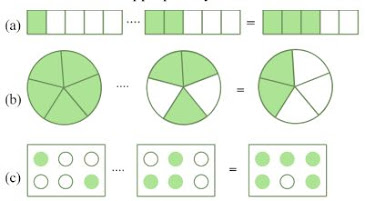Solution

(a) 1/5+2/5 = 1+2/5 = 3/5

(b) 5/5-3/5 = 5-3/5 = 2/5

(c) 2/6+3/6 = 2+3/6 = 5/6

Question 2: Solve:

(a) 1/18+1/18

(b) 8/15+3/15

(c) 7/7-5/7

(d) 1/22+21/22

(e) 12/15-7/15

(f) 5/8+3/8

(g) 1-2/3[1=3/3]

(h) 1/4+0/4

(i) 3-12/5

Solution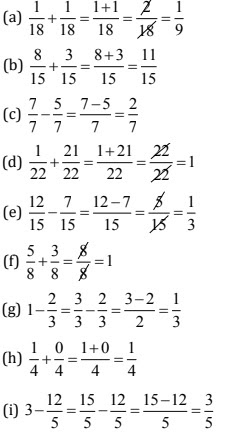Question 3: Shubham painted 2/3 of the wall space in his room. His sister Madhavi helped and painted 1/3 of the wall space. How much did they paint together?

Solution

Fraction of wall painted by Shubham = 2/3

Fraction of wall painted by Madhavi = 1/3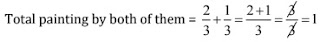Therefore, they painted complete wall.

Question 4: Fill in the missing fractions:

(a) 7/10- = 3/10

(b) -3/21 = 5/21

(c) -3/6 = 3/6

(d) ロ +5/27 = 12/27

Solution

(a) 4/10

(b) 8/21

(c) 6/6

(d) 7/27

Question 5: Javed was given of a basket of oranges. What fraction of oranges was left in the basket?

Solution

Total = 1

Fraction of Orange left = 1-5/7

= 7/7-5/7 = 7-5/7 = 2/7

Thus, 2/7 oranges was left in the basket.

Exercise 7.6

Question 1: Solve:

(a) 2/3+1/7

(b) 3/10+7/15

(c) 4/9+2/7

(d) 5/7+1/3

(e) 2/5+1/6

(f) 4/5+2/3

(g) 3/4-1/3

(h) 5/6-1/3

(i) 2/3+3/4+1/2

(j) 1/2+1/3+1/6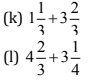(m) 16/5-7/5

(n) 4/3-1/2

Solution

(a) L.C.M. of 3 and 7 is 21

∴ 2/3+1/7 = 2×7+1×3/21 = 14+3/21 = 17/21

(b) L.C.M. of 10 and 15 is 30

∴ 3/10+7/15 = 3×3+7×2/30 = 9+14/30 = 23/30

(c) L.C.M. of 9 and 7 is 63

∴ 4/9+2/7 = 4×7+2×9/63 = 28+18/63 = 46/63

(d) L.C.M. of 7 and 3 is 21

∴ 5/7+1/3 = 5×3+7×1/21 = 15+7/21 = 22/21 =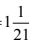(e) L.C.M. of 5 and 6 is 30

∴ 2/5+1/6 = 2×6+5×1/30 = 12+5/30 = 17/30

(f) L.C.M. of 5 and 3 is 15

∴ 4/5+2/3 = 4×3+2×5/15 = 12+10/15 = 22/15 =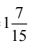(g) L.C.M. of 4 and 3 is 12

∴ 3/4-1/3 = 3×3-4×1/12 = 9-4/12 = 5/12

(h) L.C.M. of 6 and 3 is 6

∴ 5/6-1/3 = 5×1-2×1/6 = 5-2/6 =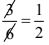(i) L.C.M. of 3, 4 and 2 is 12

∴ 2/3+3/4+1/2 = 2×4+3×3+1×6/12 = 6+9+6/12 = 23/12 =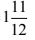(j) L.C.M. of 2, 3, and 6 is 6

∴ 1/2+1/3+1/6 = 1×3+1×2+1×1/6 = 3+2+1/6 =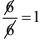(k) L.C.M. of 3 and 3 is 3

∴ 4/3+11/3 = 4+11/3 =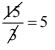(l) L.C.M. of 3 and 4 is 12

∴ 14/3+13/4 = 14×4+13×3/12 = 56+39/12 = 95/12 =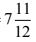(m) L.C.M. of 5 and 5 is 5

∴ 16/5-7/5 = 16-7/5 = 9/5 =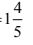(n) L.C.M. of 3 and 2 is 6

∴ 4/3-1/2 = 4×2-1×3/6 = 8-3/6 = 5/6

Question 2: Sarika bought 2/5 meter of ribbon and Lalita 3/4 meter of ribbon. What is the total length of the ribbon they bought?

Solution

Ribbon bought by Sarita = 2/5 m and Ribbon bought by Lalita = 3/4 m

Total length of ribbon = 2/5+3/4

= 2×4+5×3/20 [∵ L.C.M. of 5 and 4 is 20]

= 8+15/20 = 23/20 =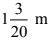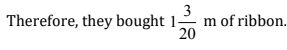Question 3: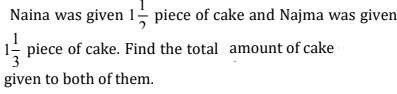Solution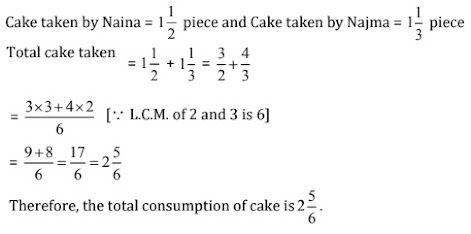Question 4: Fill in the boxes:

(a) -5/8 = 1/4

(b) -1/5 = 1/2

(c) 1/2- = 1/6

Solution

(a) 1/4+5/8 = 2+5/8 = 7/8

(b)1/2+1/5 = 5+2/10 = 7/10

(c) 1/2-1/6 = 3-1/6 = 2/6

Question 5: Complete the addition – subtraction box: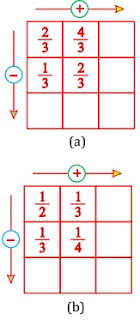Solution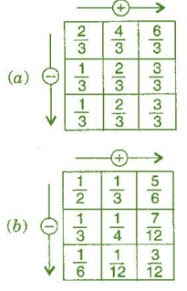Question 6: A piece of wire 7/8 meter long broke into two pieces. One piece was 1/4 meter long. How long is the other piece?

Solution

Total length of wire = 7/8 meter

Length of first part = 1/4 meter

Remaining part = 7/8-1/4 = 7×1-2×1/8  [∵ L.C.M. of 8 and 4 is 8]

=7-2/8 = 5/8 meter

Therefore, the length of remaining part is 5/8 meter.

Question 7: Nandini house is 9/10 km from her school. She walked some distance and then took a bus for 1/2 km to reach the school. How far did she walk?

Solution

Total distance between school and house = 9/10 km

Distance covered by bus = 1/2 km

Remaining distance = 9/10-1/2 = 9×1-1×5/10  [∵ L.C.M. of 10 and 2 is 10]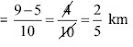Therefore, the distance covered by walking us 2/5 km

Question 8: Asha and Samuel have bookshelves of the same size partly filled with books. Asha’s shelf Is 5/6th full and Samuel’s shelf is 2/5th full. Whose bookshelf is more full? By what fraction?

Solution

5/6 and 2/5

= 5/6×5/5 = 25/30 and 2/5×6/6 = 12/30  [∵ L.C.M. of 6 and 5 is 30]

∵ 25/30 > 12/30

= 5/6 > 2/5

∴ Asha’s bookshelf is more covered than Samueal.

Difference = 25/30-12/30 = 13/30

Question 9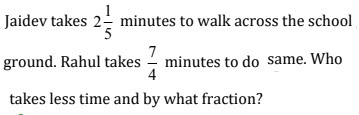Solution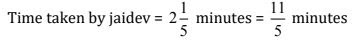Time taken by Rahul = 7/4 minutes

Difference = 11/5-7/4 = 11×4-7×5/20  [∵ L.C.M. of 5 and 4 is 20]

= 44-35/20 = 9/20 minutes

Thus, Rahul takes less time, which is 9/20 minutes.